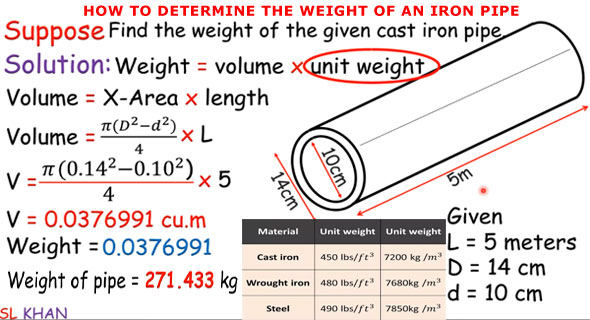How to calculate the weight of Reinforced concrete Hume pipe

# How to calculate the weight of Reinforced concrete Hume pipeHello Civil Engineers, are you confused that what Hume Pipe is. If you have doubts, this article quite helpful for you. In this article I have explained how to calculate the weight of Reinforced Hume Pipe.

RCC Hume Pipes are used for culverts , irrigation, drain and Sewer Lines.The Various Diameter of RCC Hume pipes are available such as 100 mm, 150 mm ,200 mm,250mm,300mm, 350mm,400mm,450mm, etc. RCC Hume pies is Placed across the culverts ,drainage,sewer,Irrigation for easily drain the water. This pipes are very much difficulty in installation and acquire heavy equipment for movement and placing of Hume pipes.

RCC Hume pipe have some advantage and disadvantage. These Hume pipes area much better than the metal pipes because in metal pipes because in metal pipes there will be more chances of rusting. Precast Concrete pipes may not be suitable in acidic, alkaline and temporary hard water But Reinforced concrete Hume pipes are useful for black cotton soils.
In this article I have explained how to calculate the weight of RCC Hume Pipe

## Weight of Reinforced Concrete Hume Pipe

Let us consider,
Inner radius of Hume pipes (R1) = 20 cm = 0.2 m
Outer Radius of Hume Pipe (R2) =25 cm =0.25 m
Length of RCC Hume pipe (L)    = 1 m
Density of Reinforced Cement Concrete = 2500 kg/ m3
Volume of R CC concrete Pipe = ?

We know that

Volume of outer radius =π r12 x L
Volume of Inner radius = π r22 x L

So Total Volume of RCC Hume Pipe  = π x (r22 - r12 )x L
= π x [ (0.25)2 – (0.2)2] x 1  = 0.07068 m3.

Now, Let us calculate the Weight of Reinforced Concrete Hume Pipes
So Weight of Hume Pipes = Volume of Hume Pipes x Density of Material used
= 0.07068 x 2500 = 176.714 kg.
Hence , weight of  R CC Hume pipes is 176.714 kg.

Source

Continue Reading about the "How to calculate the weight of Reinforced concrete Hume pipe" on the next page below